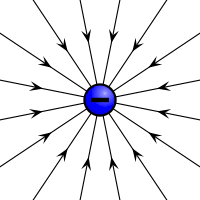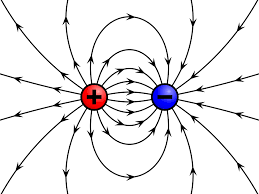## How to Calculate and Solve for the Current, Time and Quantity of Charge of an Electrolysis | The Calculator EncyclopediaThe image above represents the quantity of charge.

To compute for the quantity of charge, two essential parameters are needed and these parameters are current (I) and time (T).

The formula for calculating the quantity of charge:

Q = It

Where;
Q = Quantity of charge
I = Current
T = Time

Let’s solve an example;
Find the quantity of charge with a current of 24 and time of 12.

This implies that;
I = Current = 24
T = Time = 12

Q = It
Q = 24 x 12
Q = 288

Therefore, the quantity of charge is 288 Coulombs (C).

Calculating the Current (I) using the Quantity of Charge and Time.

I = Q / t

Where;
I = Current
Q = Quantity of charge
T = Time

Let’s solve an example;
Given that the quantity of charge is 240 with a time of 14. Find the Current?

This implies that;
Q = Quantity of charge = 240
T = Time = 14

I = Q / t
I = 240 / 14
I = 17.14

Therefore, the current is 17.14 ampere.

## How to Calculate and Solve for the Current, Time and Quantity of Charge | Nickzom CalculatorThe image above represents the quantity of charge.

To compute the quantity of charge, two essential parameters are needed and the parameters are current (I) and time (t).

The formula for calculating the quantity of charge;

Q = It

Where;
Q = Quantity of Charge
I = Current
t = Time

Let’s solve an example;
Find the quantity of charge when the current (I) is 24 amp with a time of 8 secs.

This implies that;
I = Current = 24 amp
t = Time = 8 secs

Q = It
Q = 24 x 8
Q = 192

Therefore, the quantity of charge is 192 coulombs (C).

Calculating the Current (I) of a charge using the Quantity of Charge (Q) and the time (t).

I = Q / t

Where;
I = Current
Q = Quantity of the charge
t = Time

Let’s solve an example;
Find the current of a charge with the quantity of the charge as 150 coulombs (C) and time as 15 secs.

This implies that;
Q = Quantity of the charge = 150 C
t = Time = 15 secs

I = Q / t
I = 150 / 15
I = 10

Therefore. the current is 10 Ampere (A).